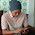# Calculation polar form into rectangular form of a complex numbers

After understanding the manual calculation method to convert complex numbers rectangular form into polar form, this time we'll learn the manual calculation method to convert complex numbers polar form into rectangular form, or the otherwise.

The complex numbers conversion's software, I have given it for free, click Converting complex numbers. It's how to compare the results of manual calculation with the execution of software's program.

Here I will give an example of the manual calculation method to convert complex numbers polar form into rectangular form, with sample questions 40 ∟ 65°

Given the polar form:
40 ∟ 65°
r (abs) = 40
φ (angle) = 65

Completion:
x (real) = r (cos φ) = 40 (cos 65) = 16,90473
y (imaginary) = r (sin φ) = 40 (sin 65) = 36,25231

Then the rectangular form becomes:
x + jy = 16,90473 + j36,25231

See a picture below, the calculation above Polar form into rectangular form

Next→
←Prev

### Choose label

1.